# NCERT Exemplar Class 10 Maths Solutions for Chapter 3 - Pair Of Linear Equations In Two Variables

## NCERT Exemplar Solutions Class 10 Maths Chapter 3 – Free PDF Download

NCERT Exemplar Class 10 Maths Chapter 3 Pair of Linear Equations in Two Variables, provided here in PDF format. Students can easily download it and prepare for the board exam. The solutions are given as per CBSE syllabus (2021-2022) followed by NCERT guidelines by our subject experts. It will help students to do the last minute revision during the exams.

Chapter 3 deals with learning how to solve the linear equation algebraically or graphically. Here, students will study different methods of solving linear equations and will be introduced with methods like elimination method, substitution method or cross multiplication method. To help students understand and solve the exercise problems available in NCERT Exemplars for Chapter 3 – pair of linear equations in two variables is given here, designed by our experts. Click here to get Exemplars for all chapters.

The topics covered in Chapter 3 for Exemplar problems and solutions are:

• Algebraic and geometrical representation of Pair of Linear Equations in Two Variables
• Graphical methods of solving a pair of Linear equations
• Algebraic methods of solving a pair of Linear equations such as substitution method, elimination method, cross-multiplication method
• Reducing the pair of equations into a linear form

Take a look at Class 10 Maths Chapter 3 NCERT Exemplar PDF below.

## Download PDF of NCERT Exemplar Solutions for Class 10 Maths Chapter 3 Pair of Linear Equations in Two Variables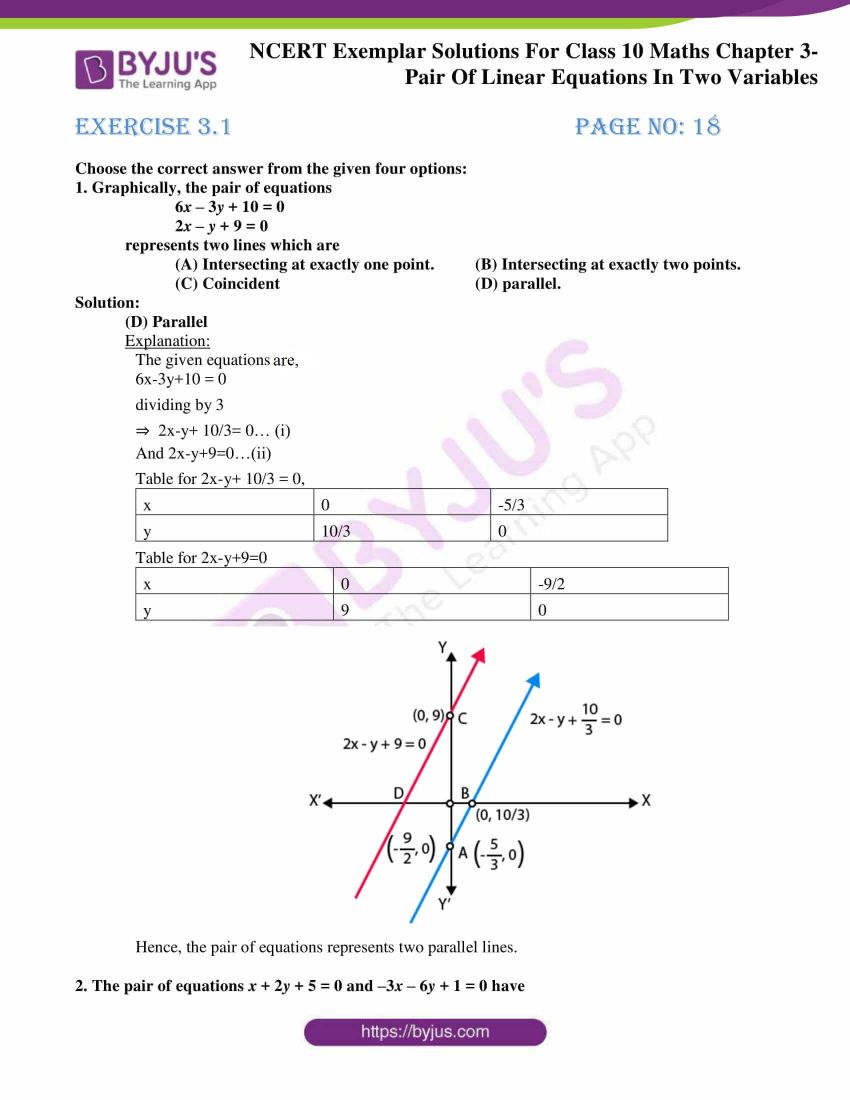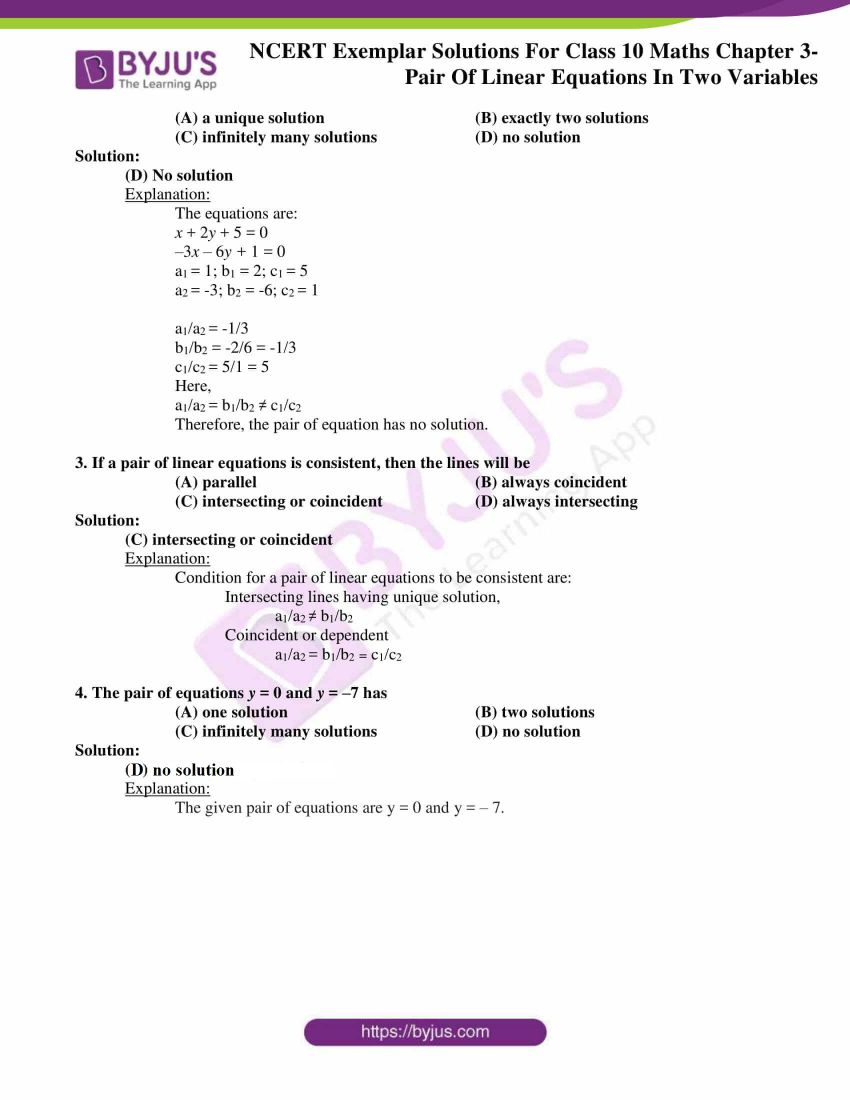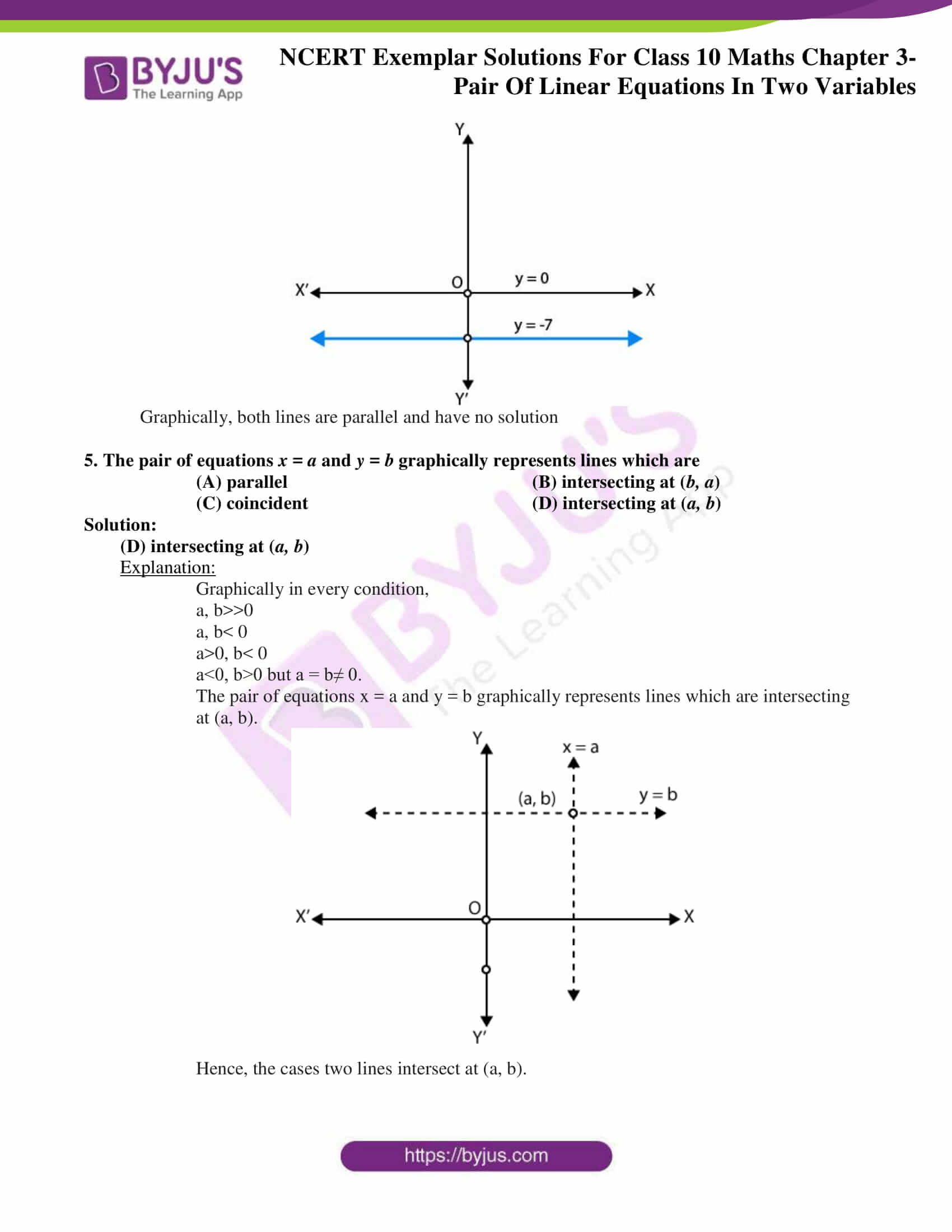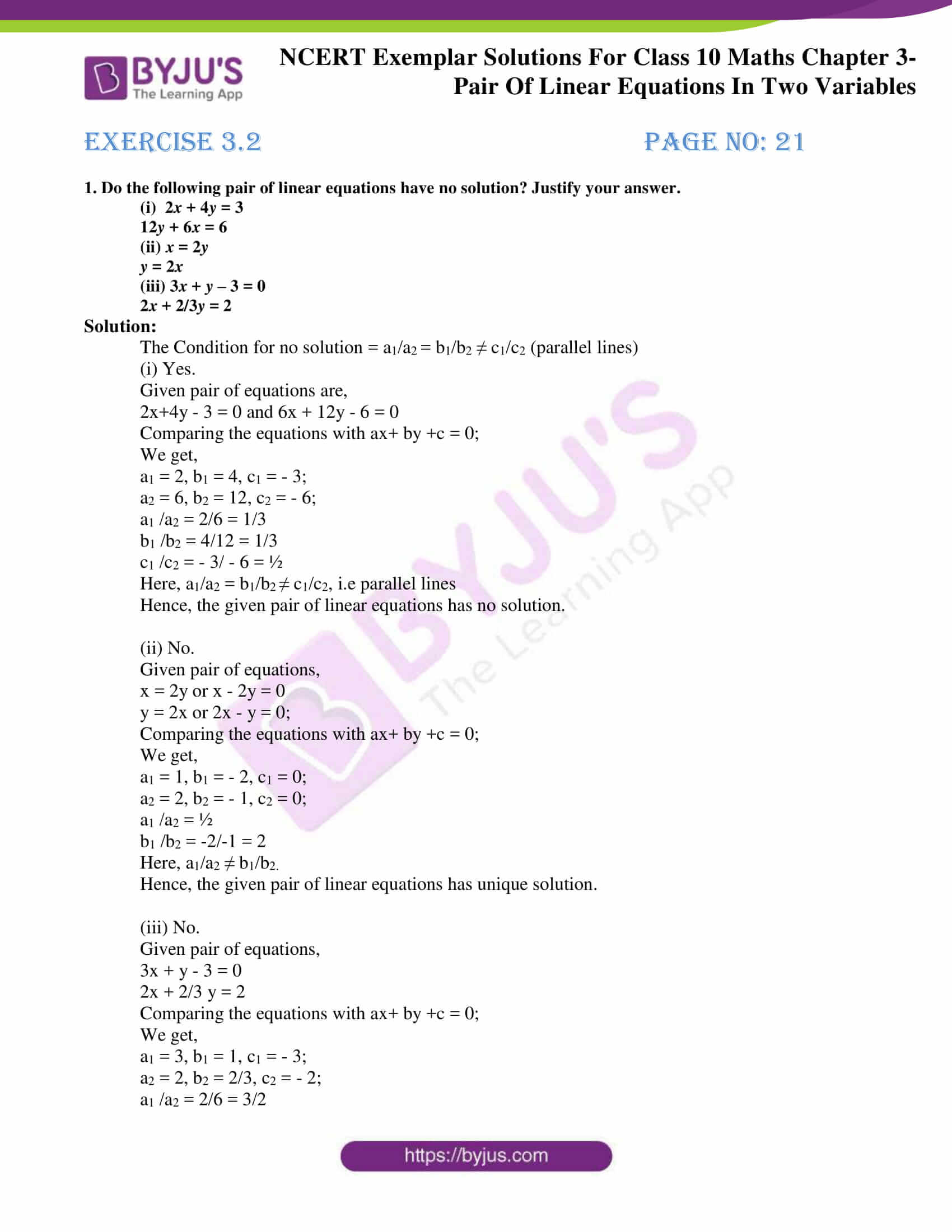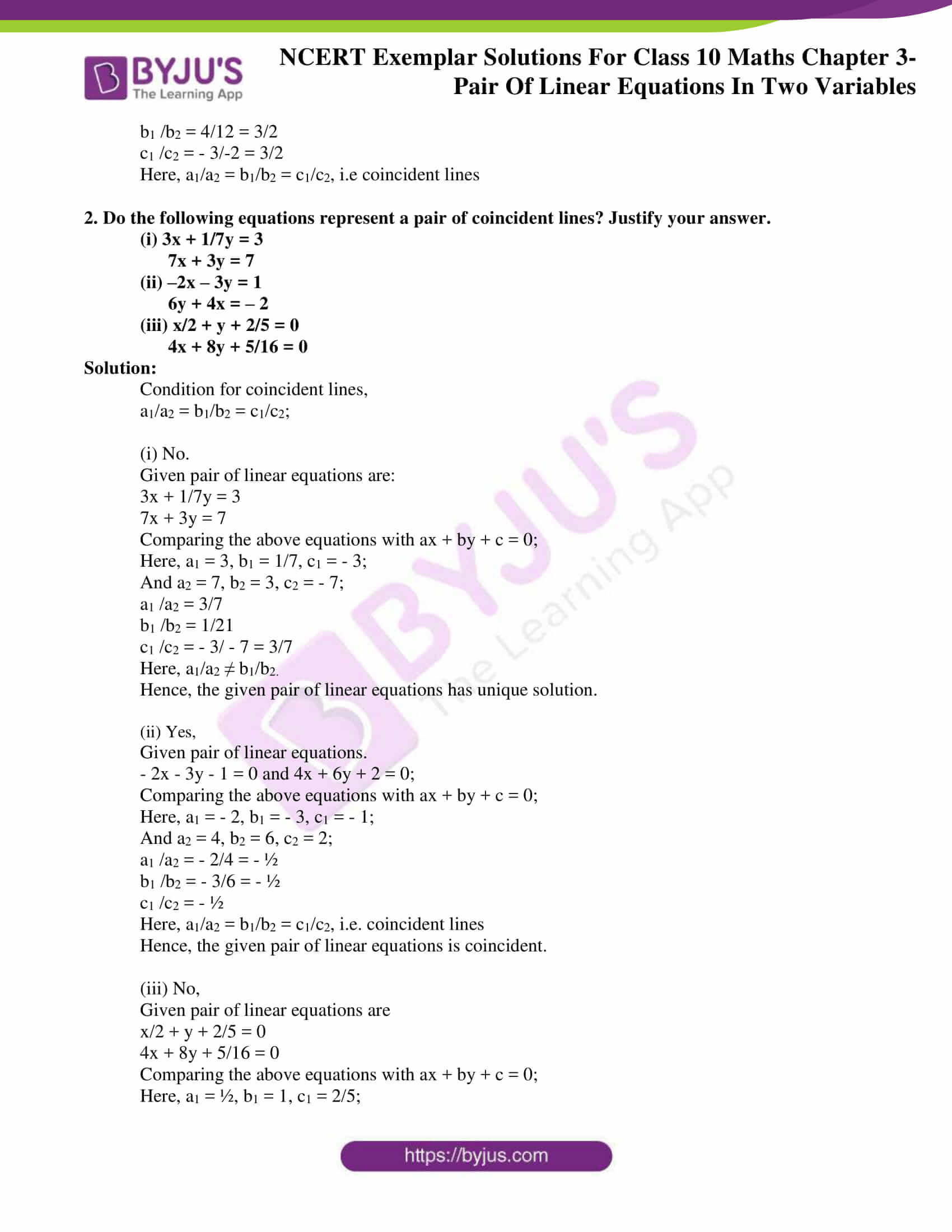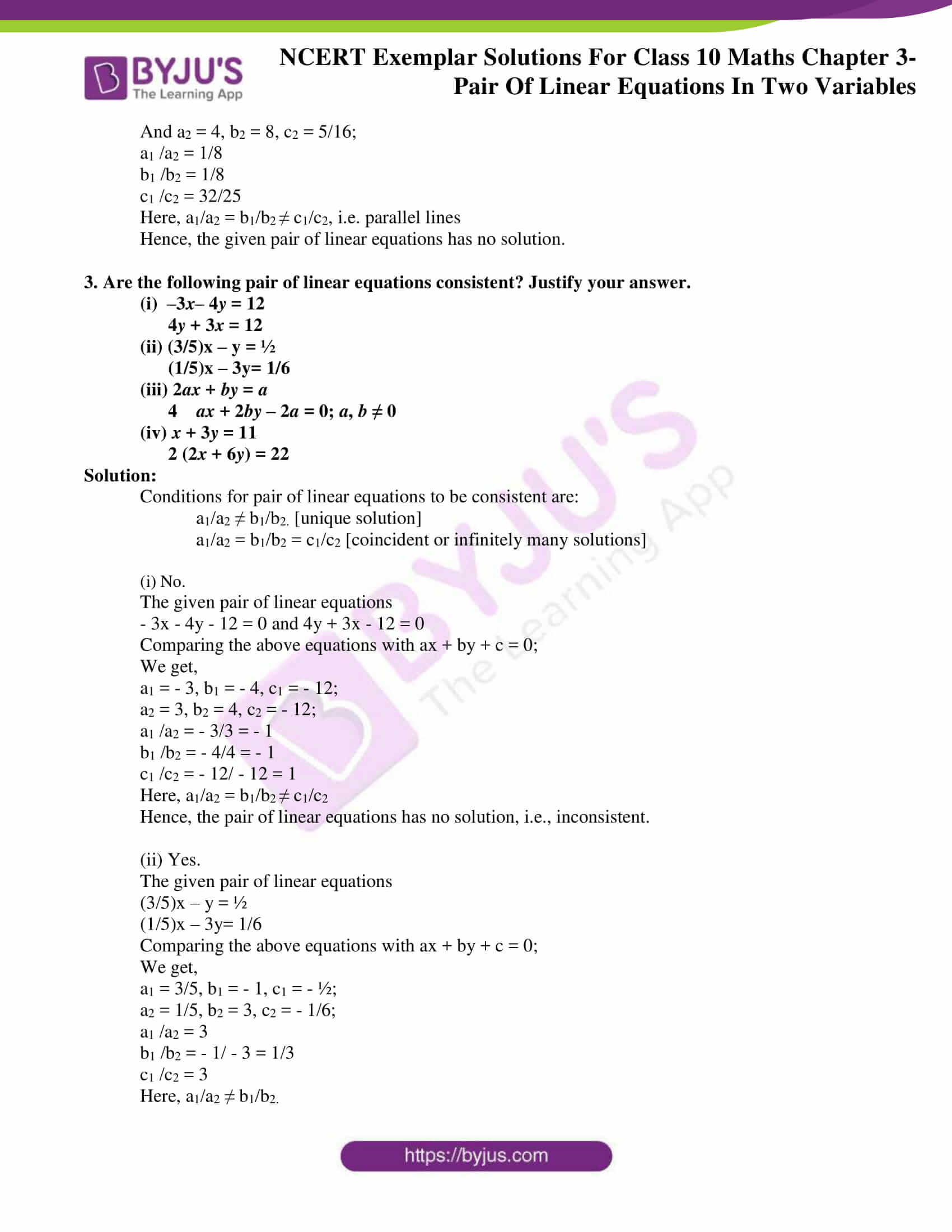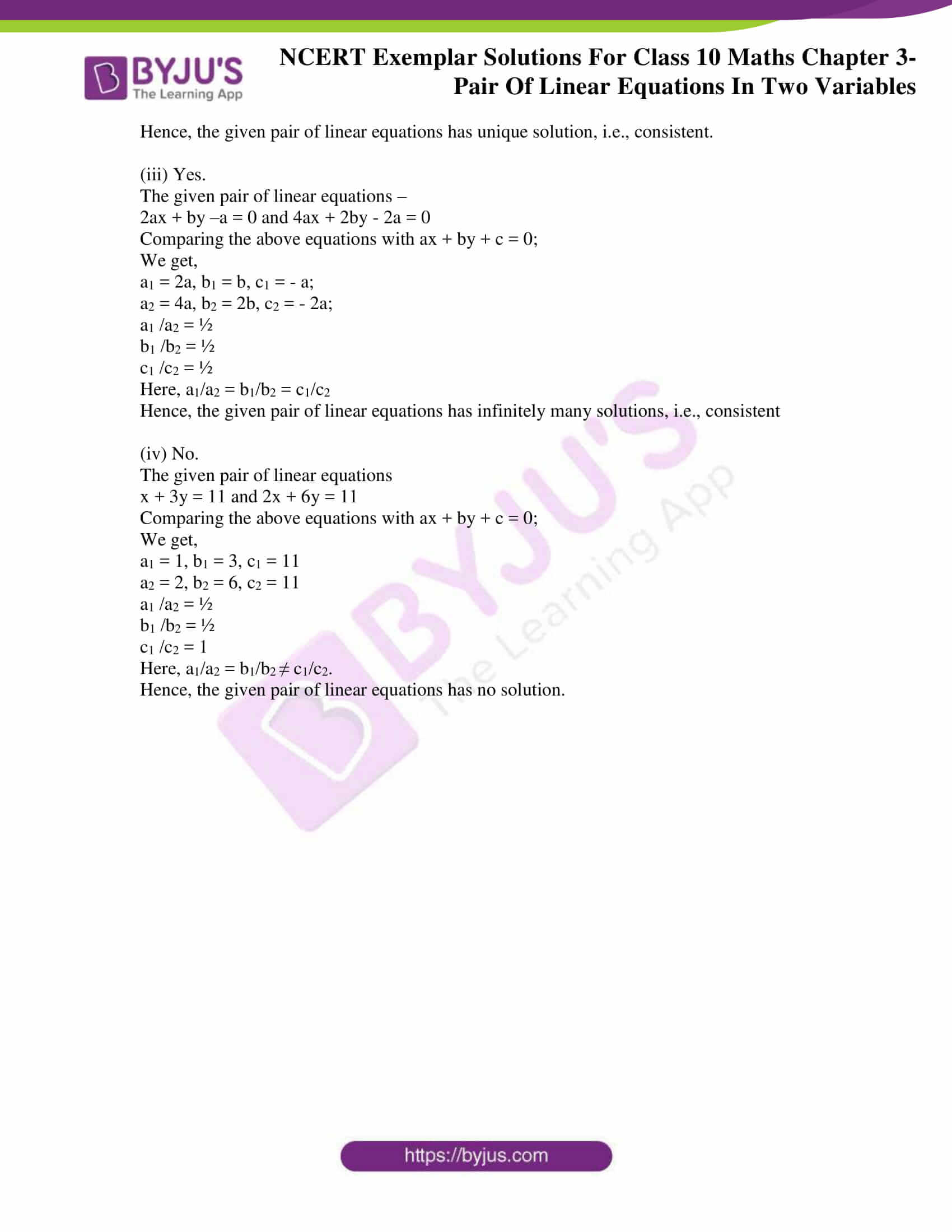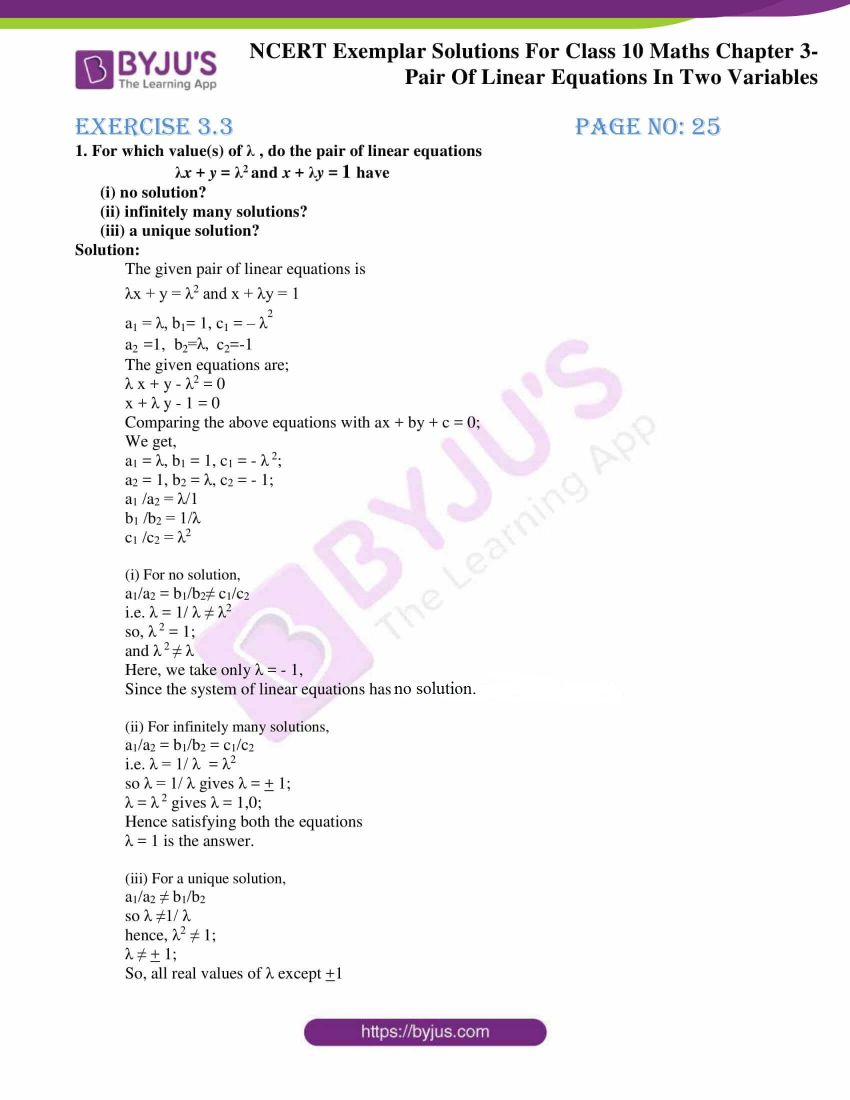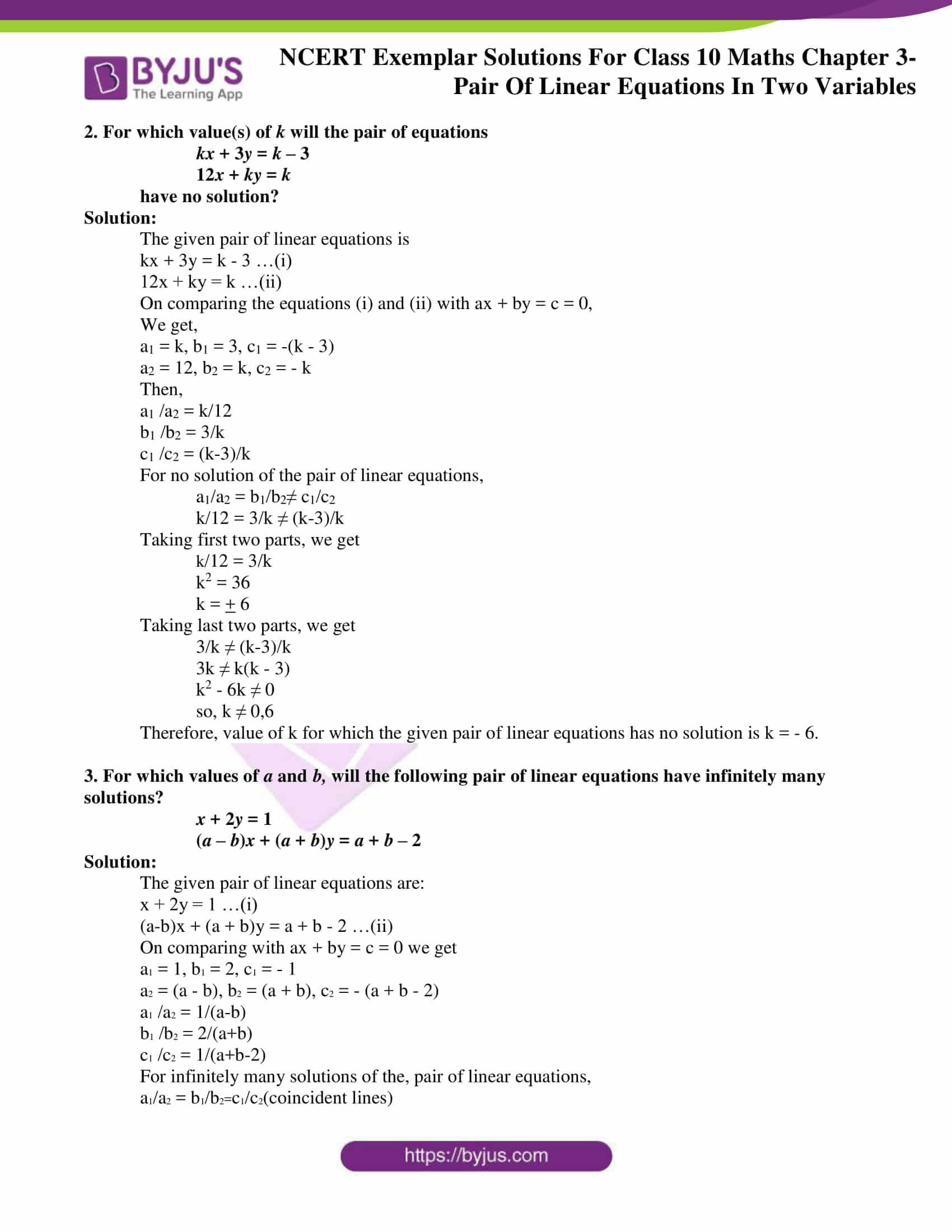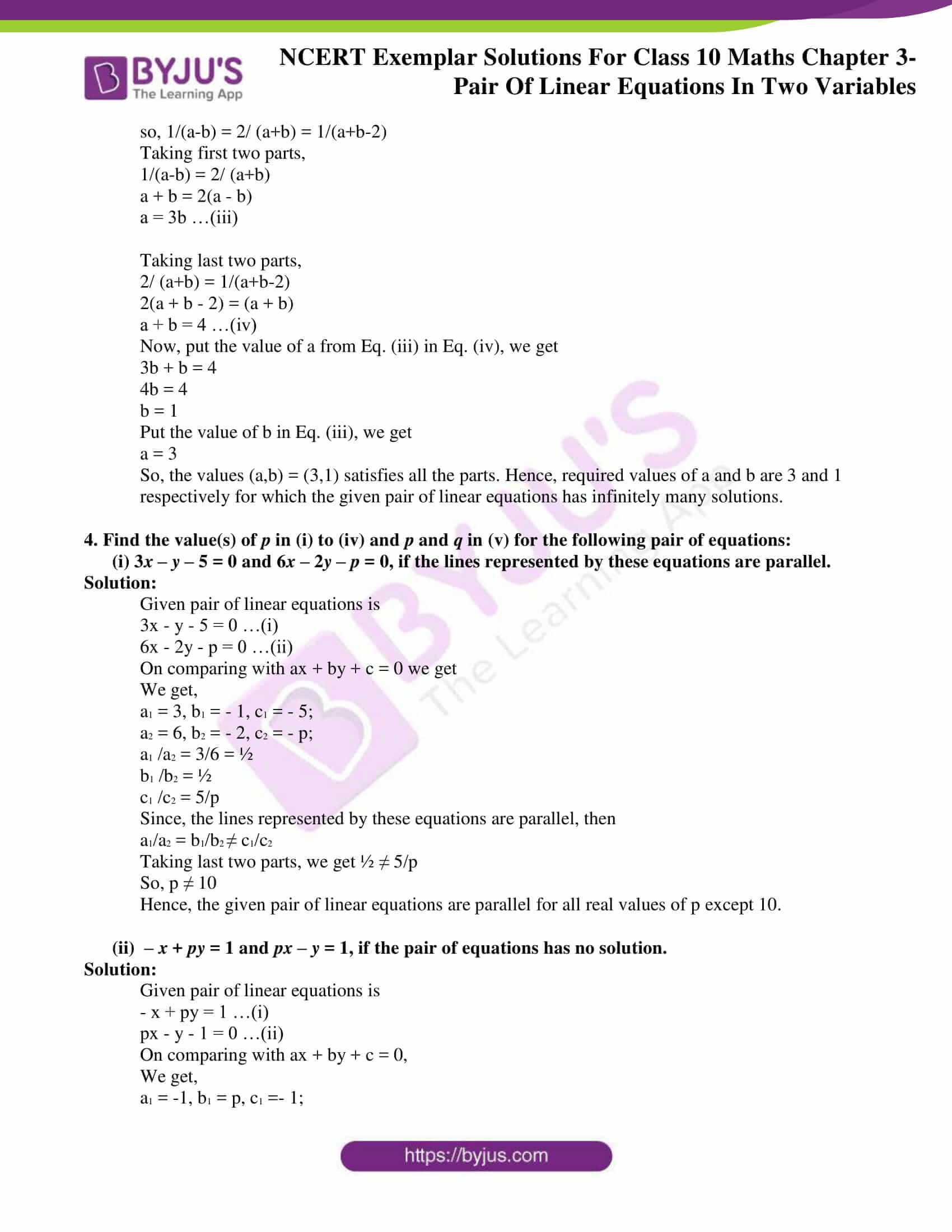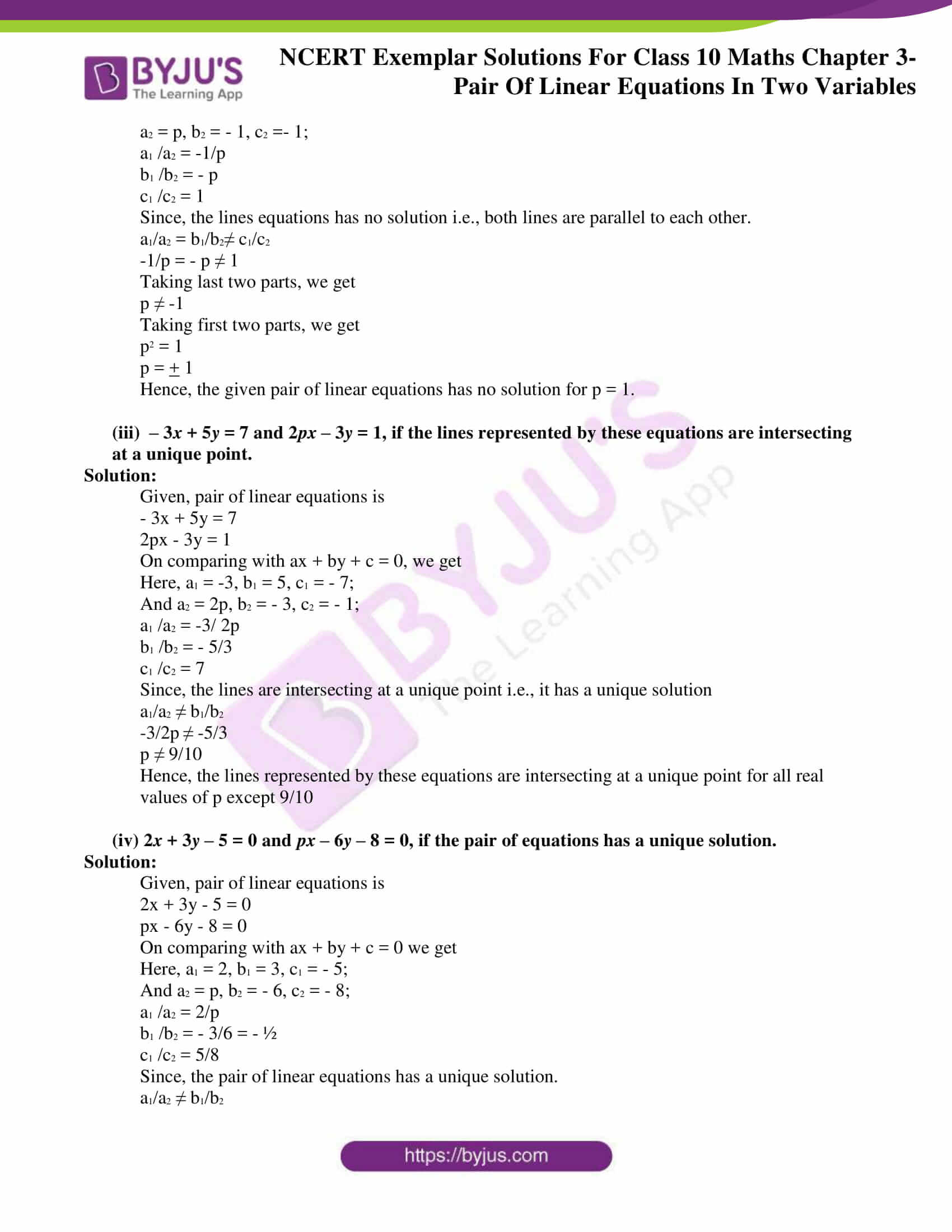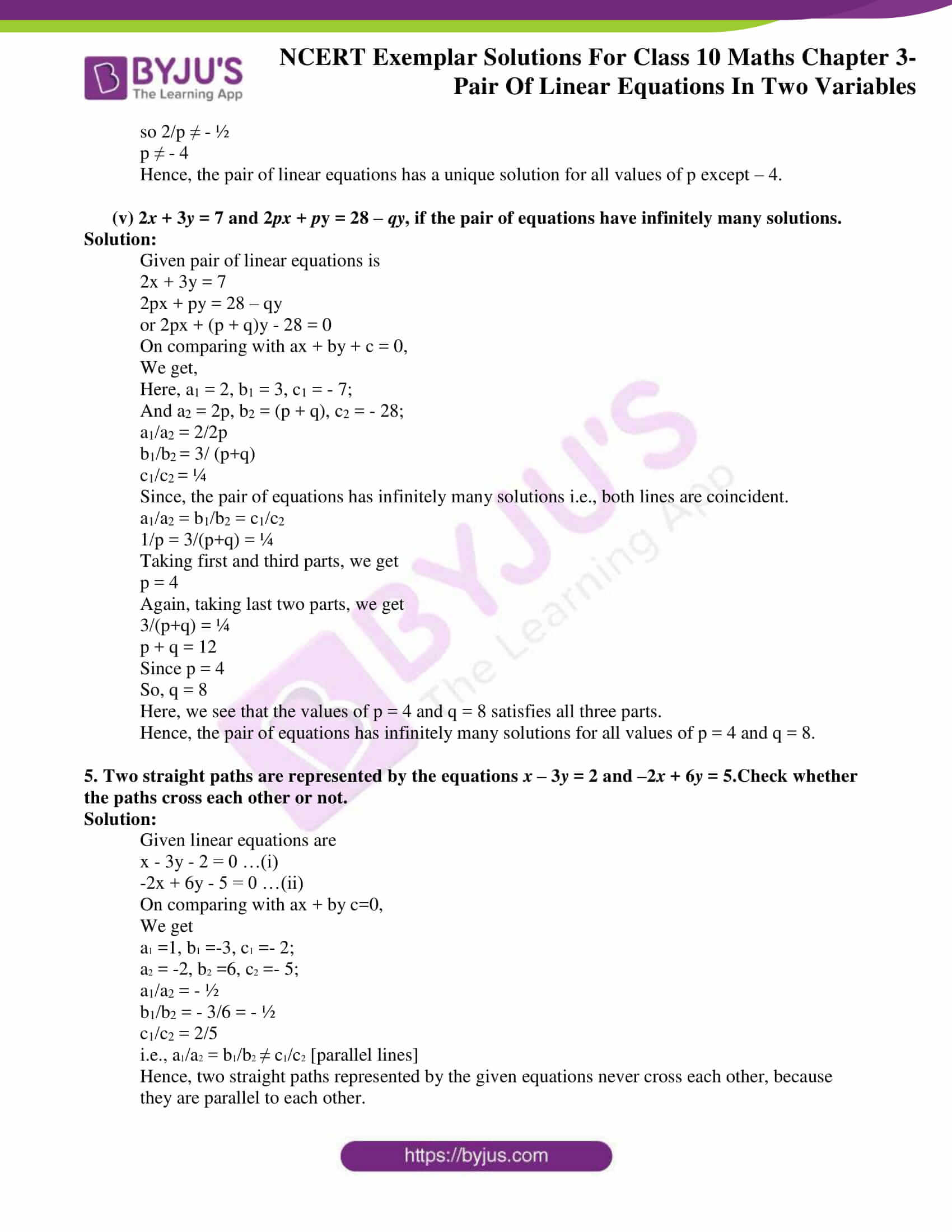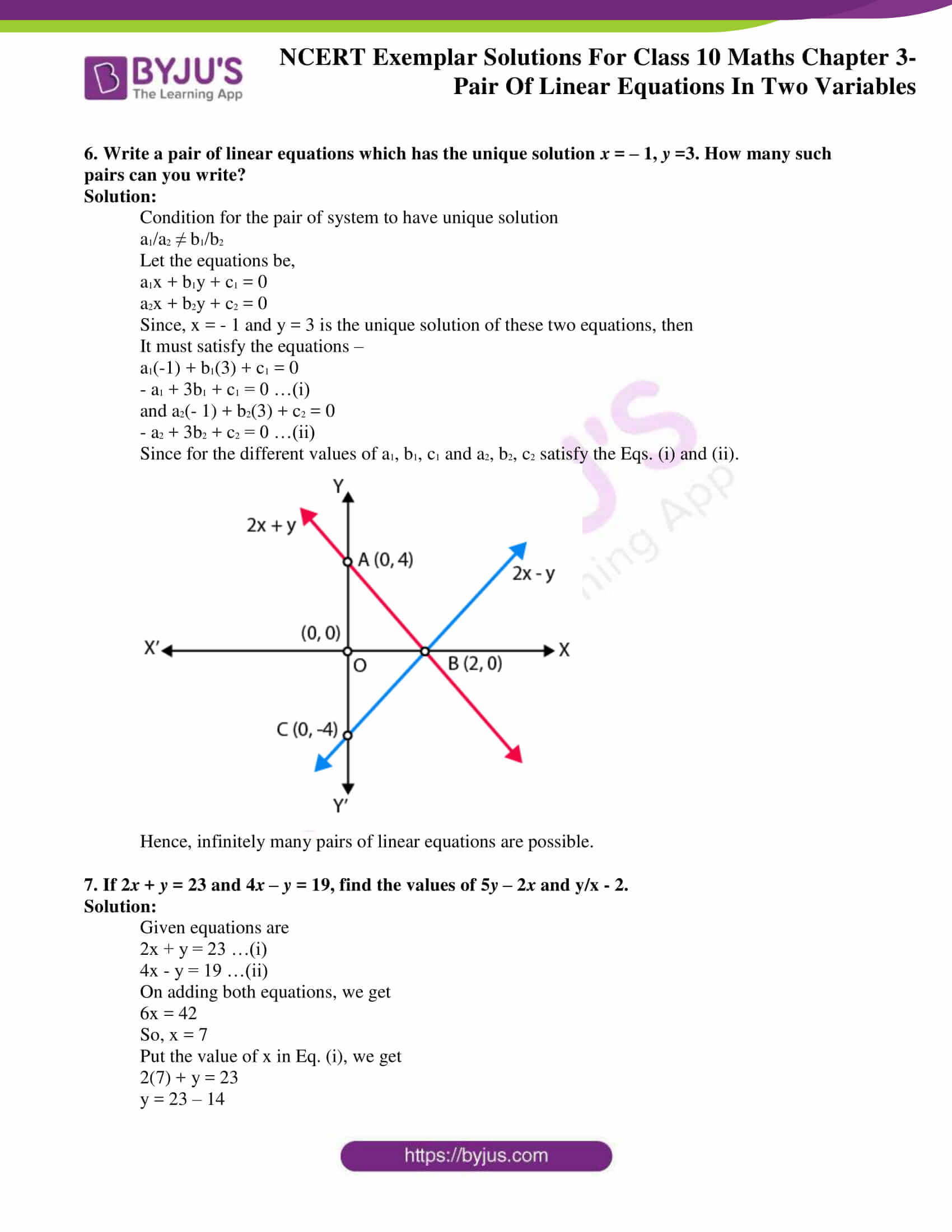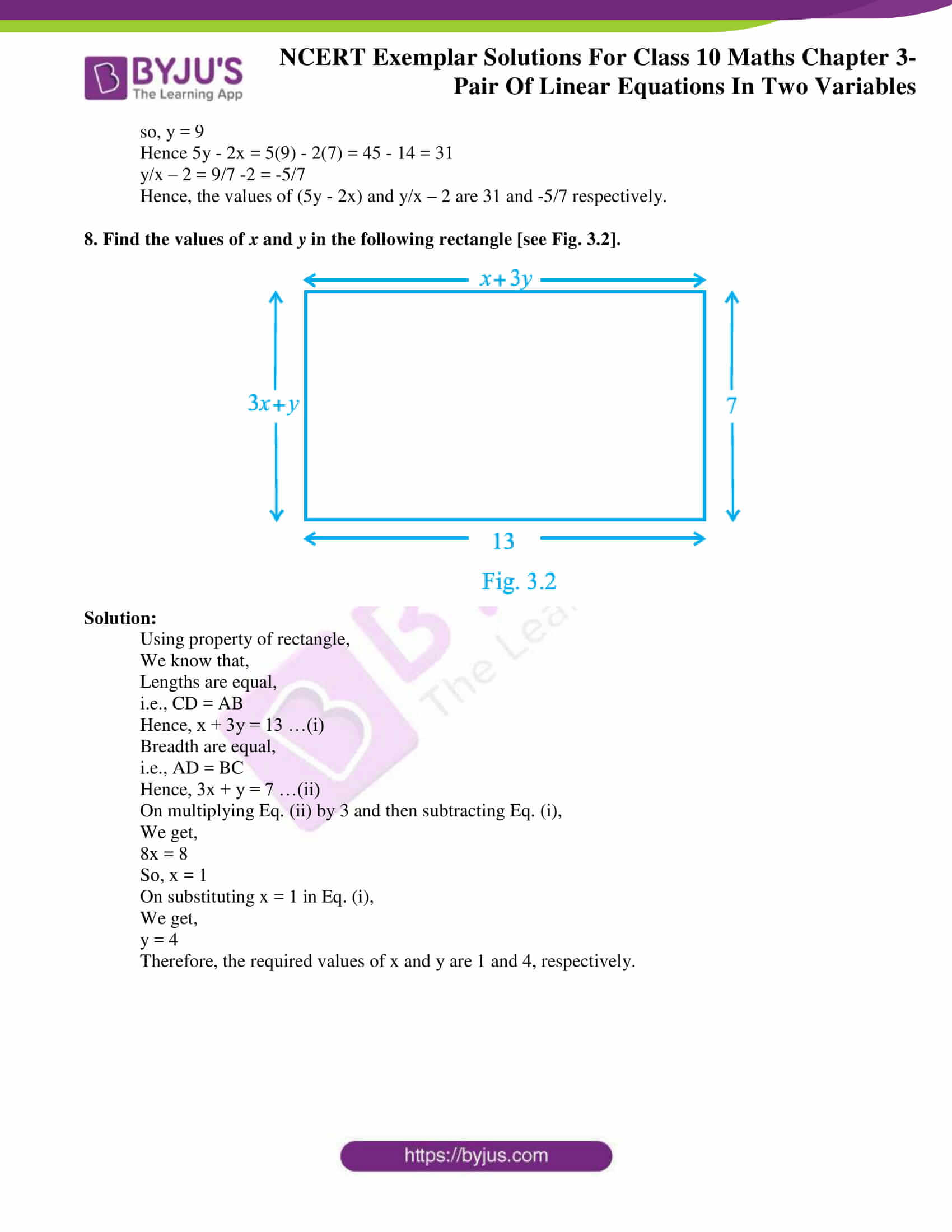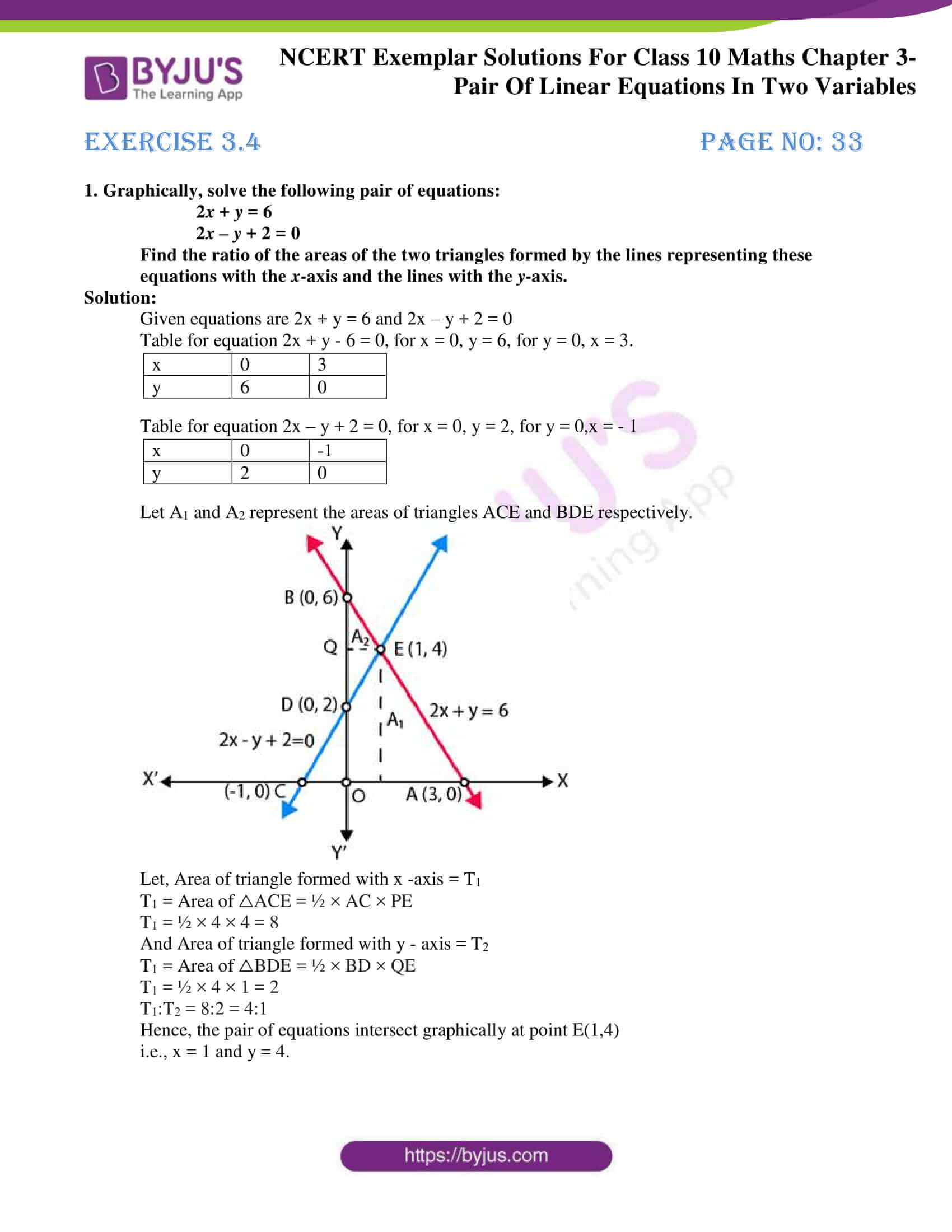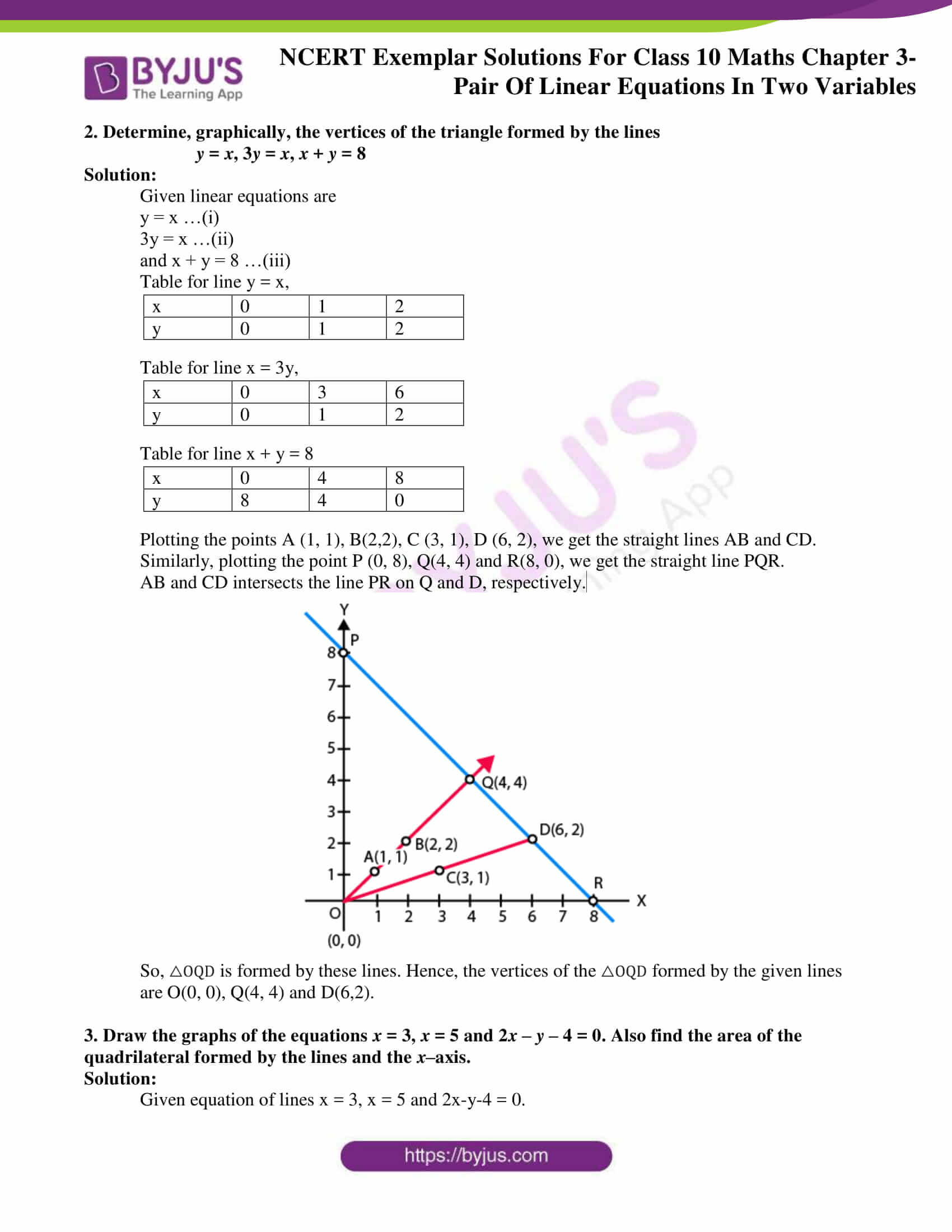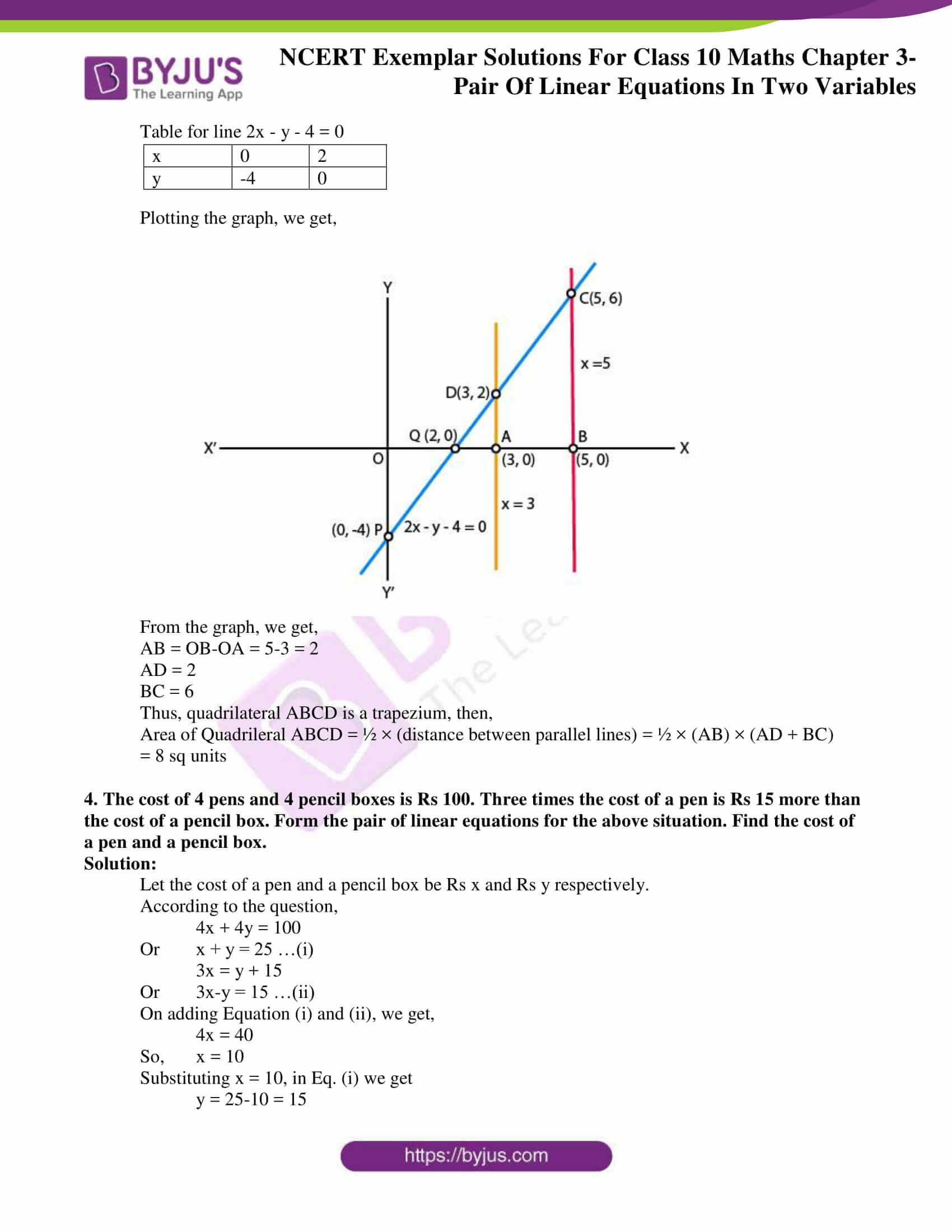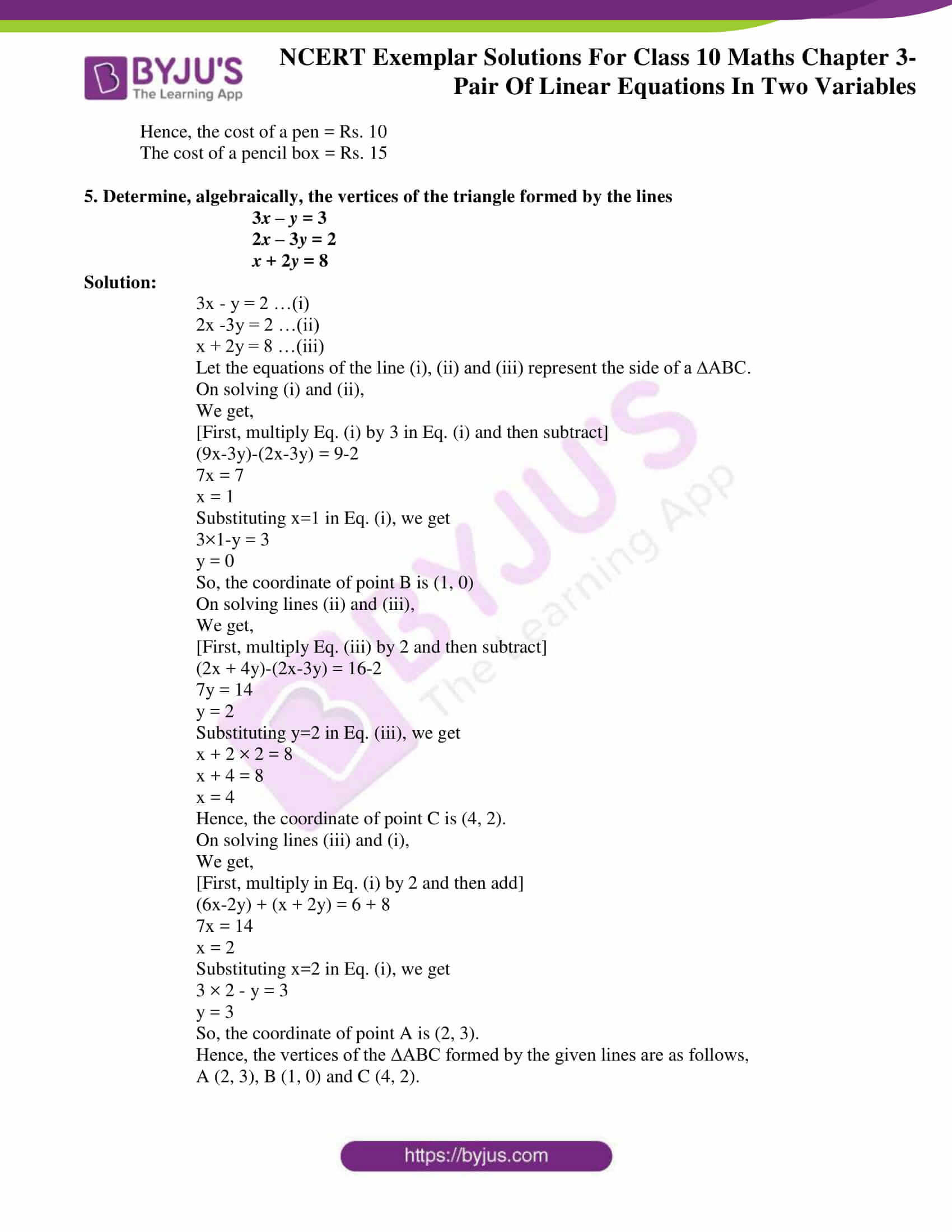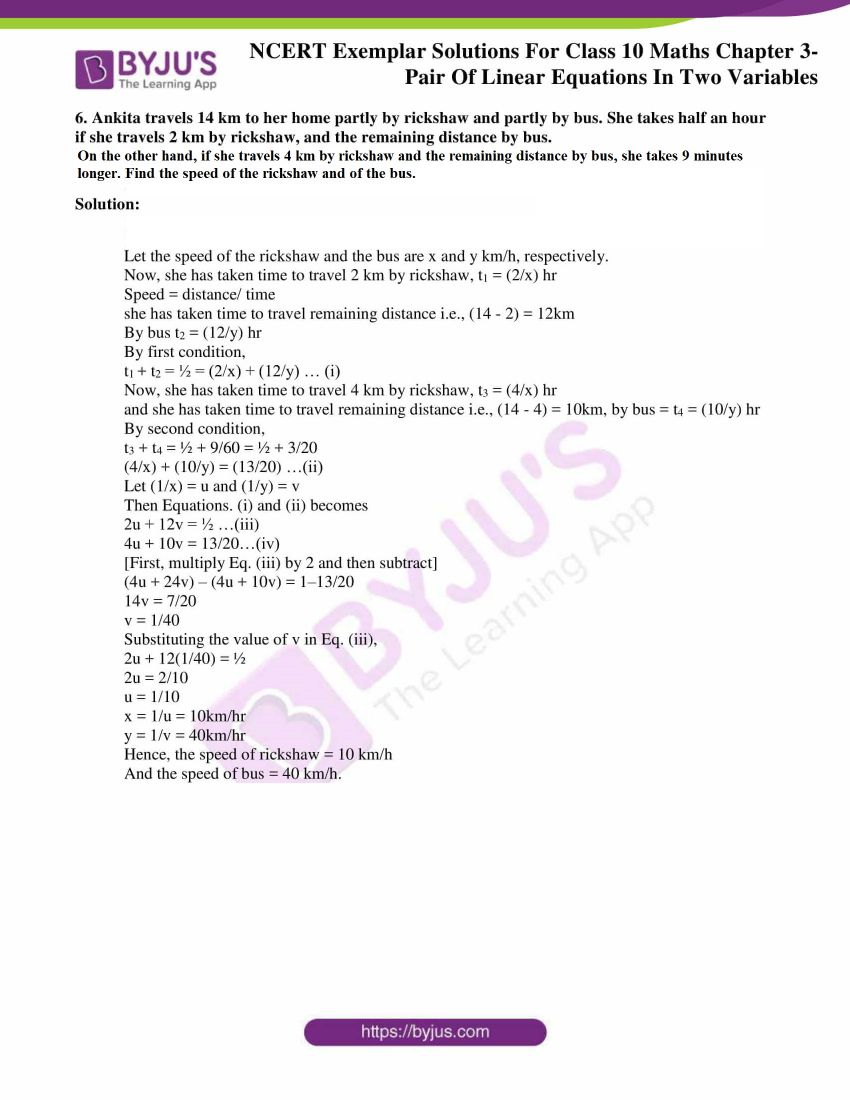## Exercise 3.1

Choose the correct answer from the given four options:

1. Graphically, the pair of equations

6x – 3y + 10 = 0

2x y + 9 = 0

represents two lines which are

(A) Intersecting at exactly one point. (B) Intersecting at exactly two points.

(C) Coincident (D) parallel.

Solution:

(D) Parallel

Explanation:

The given equations are,

6x-3y+10 = 0

dividing by 3

⇒ 2x-y+ 10/3= 0… (i)

And 2x-y+9=0…(ii)

Table for 2x-y+ 10/3 = 0,

 x 0 -5/3 y 10/3 0

Table for 2x-y+9=0

 x 0 -9/2 y 9 0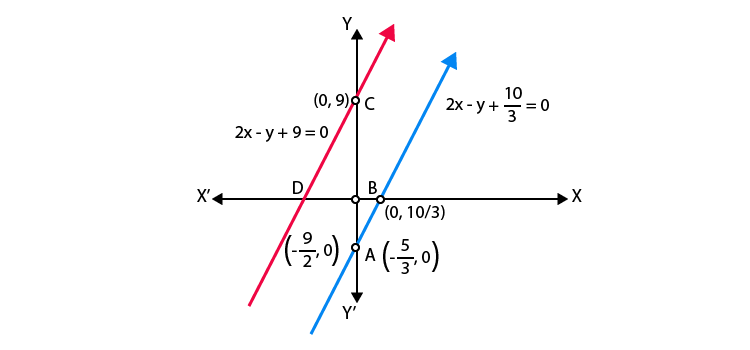Hence, the pair of equations represents two parallel lines.

2. The pair of equations x + 2y + 5 = 0 and –3x – 6y + 1 = 0 have

(A) a unique solution (B) exactly two solutions

(C) infinitely many solutions (D) no solution

Solution:

(D) No solution

Explanation:

The equations are:

x + 2y + 5 = 0

–3x – 6y + 1 = 0

a1 = 1; b1 = 2; c1 = 5

a2 = -3; b2 = -6; c2 = 1

a1/a2 = -1/3

b1/b2 = -2/6 = -1/3

c1/c2 = 5/1 = 5

Here,

a1/a2 = b1/b2 ≠ c1/c2

Therefore, the pair of equation has no solution.

3. If a pair of linear equations is consistent, then the lines will be

(A) parallel (B) always coincident

(C) intersecting or coincident (D) always intersecting

Solution:

(C) intersecting or coincident

Explanation:

Condition for a pair of linear equations to be consistent are:

Intersecting lines having unique solution,

a1/a2 ≠ b1/b2

Coincident or dependent

a1/a2 = b1/b2 = c1/c2

4. The pair of equations y = 0 and y = –7 has

(A) one solution (B) two solutions

(C) infinitely many solutions (D) no solution

Solution:

(D) no solution

Explanation:

The given pair of equations are y = 0 and y = – 7.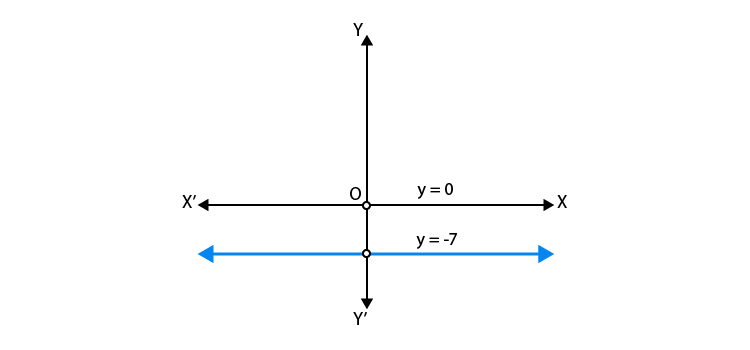Graphically, both lines are parallel and have no solution

5. The pair of equations x = a and y = b graphically represents lines which are

(A) parallel (B) intersecting at (b, a)

(C) coincident (D) intersecting at (a, b)

Solution:

(D) intersecting at (a, b)

Explanation:

Graphically in every condition,

a, b>>0

a, b< 0

a>0, b< 0

a<0, b>0 but a = b≠ 0.

The pair of equations x = a and y = b graphically represents lines which are intersecting

at (a, b).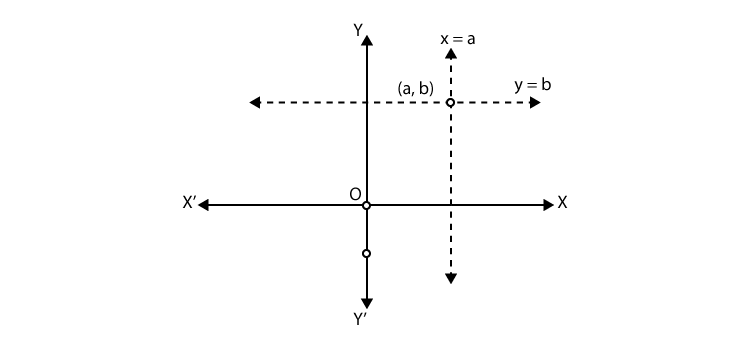Hence, the cases two lines intersect at (a, b).

## Exercise 3.2

1. Do the following pair of linear equations have no solution? Justify your answer.

(i) 2x + 4y = 3

12y + 6x = 6

(ii) x = 2y

y = 2x

(iii) 3x + y – 3 = 0

2x + 2/3y = 2

Solution:

The Condition for no solution = a1/a2 = b1/b2 ≠ c1/c2 (parallel lines)

(i) Yes.

Given pair of equations are,

2x+4y – 3 = 0 and 6x + 12y – 6 = 0

Comparing the equations with ax+ by +c = 0;

We get,

a1 = 2, b1 = 4, c1 = – 3;

a2 = 6, b2 = 12, c2 = – 6;

a1 /a2 = 2/6 = 1/3

b1 /b2 = 4/12 = 1/3

c1 /c2 = – 3/ – 6 = ½

Here, a1/a2 = b1/b2 ≠ c1/c2, i.e parallel lines

Hence, the given pair of linear equations has no solution.

(ii) No.

Given pair of equations,

x = 2y or x – 2y = 0

y = 2x or 2x – y = 0;

Comparing the equations with ax+ by +c = 0;

We get,

a1 = 1, b1 = – 2, c1 = 0;

a2 = 2, b2 = – 1, c2 = 0;

a1 /a2 = ½

b1 /b2 = -2/-1 = 2

Here, a1/a2 ≠ b1/b2.

Hence, the given pair of linear equations has unique solution.

(iii) No.

Given pair of equations,

3x + y – 3 = 0

2x + 2/3 y = 2

Comparing the equations with ax+ by +c = 0;

We get,

a1 = 3, b1 = 1, c1 = – 3;

a2 = 2, b2 = 2/3, c2 = – 2;

a1 /a2 = 2/6 = 3/2

b1 /b2 = 4/12 = 3/2

c1 /c2 = – 3/-2 = 3/2

Here, a1/a2 = b1/b2 = c1/c2, i.e coincident lines

2. Do the following equations represent a pair of coincident lines? Justify your answer.

(i) 3x + 1/7y = 3

7x + 3y = 7

(ii) –2x – 3y = 1

6y + 4x = – 2

(iii) x/2 + y + 2/5 = 0

4x + 8y + 5/16 = 0

Solution:

Condition for coincident lines,

a1/a2 = b1/b2 = c1/c2;

(i) No.

Given pair of linear equations are:

3x + 1/7y = 3

7x + 3y = 7

Comparing the above equations with ax + by + c = 0;

Here, a1 = 3, b1 = 1/7, c1 = – 3;

And a2 = 7, b2 = 3, c2 = – 7;

a1 /a2 = 3/7

b1 /b2 = 1/21

c1 /c2 = – 3/ – 7 = 3/7

Here, a1/a2 ≠ b1/b2.

Hence, the given pair of linear equations has unique solution.

(ii) Yes,

Given pair of linear equations.

– 2x – 3y – 1 = 0 and 4x + 6y + 2 = 0;

Comparing the above equations with ax + by + c = 0;

Here, a1 = – 2, b1 = – 3, c1 = – 1;

And a2 = 4, b2 = 6, c2 = 2;

a1 /a2 = – 2/4 = – ½

b1 /b2 = – 3/6 = – ½

c1 /c2 = – ½

Here, a1/a2 = b1/b2 = c1/c2, i.e. coincident lines

Hence, the given pair of linear equations is coincident.

(iii) No,

Given pair of linear equations are

x/2 + y + 2/5 = 0

4x + 8y + 5/16 = 0

Comparing the above equations with ax + by + c = 0;

Here, a1 = ½, b1 = 1, c1 = 2/5;

And a2 = 4, b2 = 8, c2 = 5/16;

a1 /a2 = 1/8

b1 /b2 = 1/8

c1 /c2 = 32/25

Here, a1/a2 = b1/b2 ≠ c1/c2, i.e. parallel lines

Hence, the given pair of linear equations has no solution.

3. Are the following pair of linear equations consistent? Justify your answer.

(i) –3x– 4y = 12

4y + 3x = 12

(ii) (3/5)x – y = ½

(1/5)x – 3y= 1/6

(iii) 2ax + by = a

1. ax + 2by – 2a = 0; a, b ≠ 0

(iv) x + 3y = 11

2 (2x + 6y) = 22

Solution:

Conditions for pair of linear equations to be consistent are:

a1/a2 ≠ b1/b2. [unique solution]

a1/a2 = b1/b2 = c1/c2 [coincident or infinitely many solutions]

(i) No.

The given pair of linear equations

– 3x – 4y – 12 = 0 and 4y + 3x – 12 = 0

Comparing the above equations with ax + by + c = 0;

We get,

a1 = – 3, b1 = – 4, c1 = – 12;

a2 = 3, b2 = 4, c2 = – 12;

a1 /a2 = – 3/3 = – 1

b1 /b2 = – 4/4 = – 1

c1 /c2 = – 12/ – 12 = 1

Here, a1/a2 = b1/b2 ≠ c1/c2

Hence, the pair of linear equations has no solution, i.e., inconsistent.

(ii) Yes.

The given pair of linear equations

(3/5)x – y = ½

(1/5)x – 3y= 1/6

Comparing the above equations with ax + by + c = 0;

We get,

a1 = 3/5, b1 = – 1, c1 = – ½;

a2 = 1/5, b2 = 3, c2 = – 1/6;

a1 /a2 = 3

b1 /b2 = – 1/ – 3 = 1/3

c1 /c2 = 3

Here, a1/a2 ≠ b1/b2.

Hence, the given pair of linear equations has unique solution, i.e., consistent.

(iii) Yes.

The given pair of linear equations –

2ax + by –a = 0 and 4ax + 2by – 2a = 0

Comparing the above equations with ax + by + c = 0;

We get,

a1 = 2a, b1 = b, c1 = – a;

a2 = 4a, b2 = 2b, c2 = – 2a;

a1 /a2 = ½

b1 /b2 = ½

c1 /c2 = ½

Here, a1/a2 = b1/b2 = c1/c2

Hence, the given pair of linear equations has infinitely many solutions, i.e., consistent

(iv) No.

The given pair of linear equations

x + 3y = 11 and 2x + 6y = 11

Comparing the above equations with ax + by + c = 0;

We get,

a1 = 1, b1 = 3, c1 = 11

a2 = 2, b2 = 6, c2 = 11

a1 /a2 = ½

b1 /b2 = ½

c1 /c2 = 1

Here, a1/a2 = b1/b2 ≠ c1/c2.

Hence, the given pair of linear equations has no solution.

## Exercise 3.3

1. For which value(s) of λ , do the pair of linear equations

λx + y = λ2 and x + λy = 1 have

(i) no solution?

(ii) infinitely many solutions?

(iii) a unique solution?

Solution:

The given pair of linear equations is

λx + y = λ2 and x + λy = 1

a1 = λ, b1= 1, c1 = – λ2

a2 =1, b2=λ, c2=-1

The given equations are;

λ x + y – λ2 = 0

x + λ y – 1 = 0

Comparing the above equations with ax + by + c = 0;

We get,

a1 = λ, b1 = 1, c1 = – λ 2;

a2 = 1, b2 = λ, c2 = – 1;

a1 /a2 = λ/1

b1 /b2 = 1/λ

c1 /c2 = λ2

(i) For no solution,

a1/a2 = b1/b2≠ c1/c2

i.e. λ = 1/ λ ≠ λ2

so, λ 2 = 1;

and λ 2 ≠ λ

Here, we take only λ = – 1,

Since the system of linear equations has no solution.

(ii) For infinitely many solutions,

a1/a2 = b1/b2 = c1/c2

i.e. λ = 1/ λ  = λ2

so λ = 1/ λ gives λ = + 1;

λ = λ 2 gives λ = 1,0;

Hence satisfying both the equations

λ = 1 is the answer.

(iii) For a unique solution,

a1/a2 ≠ b1/b2

so λ ≠1/ λ

hence, λ2 ≠ 1;

λ ≠ + 1;

So, all real values of λ except +1

2. For which value(s) of k will the pair of equations

kx + 3y = k – 3

12x + ky = k

have no solution?

Solution:

The given pair of linear equations is

kx + 3y = k – 3 …(i)

12x + ky = k …(ii)

On comparing the equations (i) and (ii) with ax + by = c = 0,

We get,

a1 = k, b1 = 3, c1 = -(k – 3)

a2 = 12, b2 = k, c2 = – k

Then,

a1 /a2 = k/12

b1 /b2 = 3/k

c1 /c2 = (k-3)/k

For no solution of the pair of linear equations,

a1/a2 = b1/b2≠ c1/c2

k/12 = 3/k ≠ (k-3)/k

Taking first two parts, we get

k/12 = 3/k

k2 = 36

k = + 6

Taking last two parts, we get

3/k ≠ (k-3)/k

3k ≠ k(k – 3)

k2 – 6k ≠ 0

so, k ≠ 0,6

Therefore, value of k for which the given pair of linear equations has no solution is k = – 6.

3. For which values of a and b, will the following pair of linear equations have infinitely many solutions?

x + 2y = 1

(a b)x + (a + b)y = a + b – 2

Solution:

The given pair of linear equations are:

x + 2y = 1 …(i)

(a-b)x + (a + b)y = a + b – 2 …(ii)

On comparing with ax + by = c = 0 we get

a1 = 1, b1 = 2, c1 = – 1

a2 = (a – b), b2 = (a + b), c2 = – (a + b – 2)

a1 /a2 = 1/(a-b)

b1 /b2 = 2/(a+b)

c1 /c2 = 1/(a+b-2)

For infinitely many solutions of the, pair of linear equations,

a1/a2 = b1/b2=c1/c2(coincident lines)

so, 1/(a-b) = 2/ (a+b) = 1/(a+b-2)

Taking first two parts,

1/(a-b) = 2/ (a+b)

a + b = 2(a – b)

a = 3b …(iii)

Taking last two parts,

2/ (a+b) = 1/(a+b-2)

2(a + b – 2) = (a + b)

a + b = 4 …(iv)

Now, put the value of a from Eq. (iii) in Eq. (iv), we get

3b + b = 4

4b = 4

b = 1

Put the value of b in Eq. (iii), we get

a = 3

So, the values (a,b) = (3,1) satisfies all the parts. Hence, required values of a and b are 3 and 1 respectively for which the given pair of linear equations has infinitely many solutions.

4. Find the value(s) of p in (i) to (iv) and p and q in (v) for the following pair of equations:

(i) 3x y – 5 = 0 and 6x – 2y p = 0, if the lines represented by these equations are parallel.

Solution:

Given pair of linear equations is

3x – y – 5 = 0 …(i)

6x – 2y – p = 0 …(ii)

On comparing with ax + by + c = 0 we get

We get,

a1 = 3, b1 = – 1, c1 = – 5;

a2 = 6, b2 = – 2, c2 = – p;

a1 /a2 = 3/6 = ½

b1 /b2 = ½

c1 /c2 = 5/p

Since, the lines represented by these equations are parallel, then

a1/a2 = b1/b2 ≠ c1/c2

Taking last two parts, we get ½ ≠ 5/p

So, p ≠ 10

Hence, the given pair of linear equations are parallel for all real values of p except 10.

(ii) – x + py = 1 and px y = 1, if the pair of equations has no solution.

Solution:

Given pair of linear equations is

– x + py = 1 …(i)

px – y – 1 = 0 …(ii)

On comparing with ax + by + c = 0,

We get,

a1 = -1, b1 = p, c1 =- 1;

a2 = p, b2 = – 1, c2 =- 1;

a1 /a2 = -1/p

b1 /b2 = – p

c1 /c2 = 1

Since, the lines equations has no solution i.e., both lines are parallel to each other.

a1/a2 = b1/b2≠ c1/c2

-1/p = – p ≠ 1

Taking last two parts, we get

p ≠ -1

Taking first two parts, we get

p2 = 1

p = + 1

Hence, the given pair of linear equations has no solution for p = 1.

(iii) – 3x + 5y = 7 and 2px – 3y = 1, if the lines represented by these equations are intersecting at a unique point.

Solution:

Given, pair of linear equations is

– 3x + 5y = 7

2px – 3y = 1

On comparing with ax + by + c = 0, we get

Here, a1 = -3, b1 = 5, c1 = – 7;

And a2 = 2p, b2 = – 3, c2 = – 1;

a1 /a2 = -3/ 2p

b1 /b2 = – 5/3

c1 /c2 = 7

Since, the lines are intersecting at a unique point i.e., it has a unique solution

a1/a2 ≠ b1/b2

-3/2p ≠ -5/3

p ≠ 9/10

Hence, the lines represented by these equations are intersecting at a unique point for all real values of p except 9/10

(iv) 2x + 3y – 5 = 0 and px – 6y – 8 = 0, if the pair of equations has a unique solution.

Solution:

Given, pair of linear equations is

2x + 3y – 5 = 0

px – 6y – 8 = 0

On comparing with ax + by + c = 0 we get

Here, a1 = 2, b1 = 3, c1 = – 5;

And a2 = p, b2 = – 6, c2 = – 8;

a1 /a2 = 2/p

b1 /b2 = – 3/6 = – ½

c1 /c2 = 5/8

Since, the pair of linear equations has a unique solution.

a1/a2 ≠ b1/b2

so 2/p ≠ – ½

p ≠ – 4

Hence, the pair of linear equations has a unique solution for all values of p except – 4.

(v) 2x + 3y = 7 and 2px + py = 28 – qy, if the pair of equations have infinitely many solutions.

Solution:

Given pair of linear equations is

2x + 3y = 7

2px + py = 28 – qy

or 2px + (p + q)y – 28 = 0

On comparing with ax + by + c = 0,

We get,

Here, a1 = 2, b1 = 3, c1 = – 7;

And a2 = 2p, b2 = (p + q), c2 = – 28;

a1/a2 = 2/2p

b1/b2 = 3/ (p+q)

c1/c2 = ¼

Since, the pair of equations has infinitely many solutions i.e., both lines are coincident.

a1/a2 = b1/b2 = c1/c2

1/p = 3/(p+q) = ¼

Taking first and third parts, we get

p = 4

Again, taking last two parts, we get

3/(p+q) = ¼

p + q = 12

Since p = 4

So, q = 8

Here, we see that the values of p = 4 and q = 8 satisfies all three parts.

Hence, the pair of equations has infinitely many solutions for all values of p = 4 and q = 8.

5. Two straight paths are represented by the equations x – 3y = 2 and –2x + 6y = 5.Check whether the paths cross each other or not.

Solution:

Given linear equations are

x – 3y – 2 = 0 …(i)

-2x + 6y – 5 = 0 …(ii)

On comparing with ax + by c=0,

We get

a1 =1, b1 =-3, c1 =- 2;

a2 = -2, b2 =6, c2 =- 5;

a1/a2 = – ½

b1/b2 = – 3/6 = – ½

c1/c2 = 2/5

i.e., a1/a2 = b1/b2 ≠ c1/c2 [parallel lines]

Hence, two straight paths represented by the given equations never cross each other, because

they are parallel to each other.

6. Write a pair of linear equations which has the unique solution x = – 1, y =3. How many such pairs can you write?

Solution:

Condition for the pair of system to have unique solution

a1/a2 ≠ b1/b2

Let the equations be,

a1x + b1y + c1 = 0

a2x + b2y + c2 = 0

Since, x = – 1 and y = 3 is the unique solution of these two equations, then

It must satisfy the equations –

a1(-1) + b1(3) + c1 = 0

– a1 + 3b1 + c1 = 0 …(i)

and a2(- 1) + b2(3) + c2 = 0

– a2 + 3b2 + c2 = 0 …(ii)

Since for the different values of a1, b1, c1 and a2, b2, c2 satisfy the Eqs. (i) and (ii).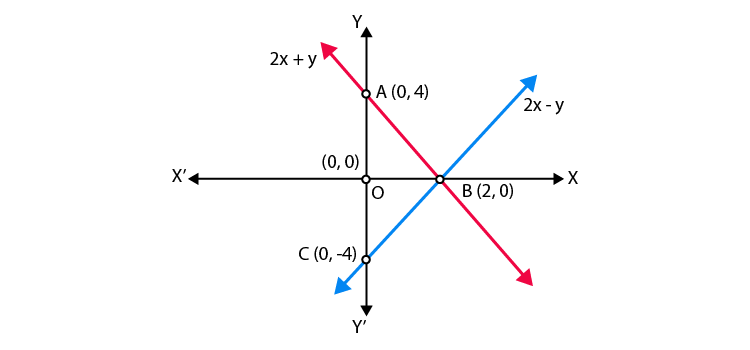Hence, infinitely many pairs of linear equations are possible.

7. If 2x + y = 23 and 4x y = 19, find the values of 5y – 2x and y/x – 2.

Solution:

Given equations are

2x + y = 23 …(i)

4x – y = 19 …(ii)

On adding both equations, we get

6x = 42

So, x = 7

Put the value of x in Eq. (i), we get

2(7) + y = 23

y = 23 – 14

so, y = 9

Hence 5y – 2x = 5(9) – 2(7) = 45 – 14 = 31

y/x – 2 = 9/7 -2 = -5/7

Hence, the values of (5y – 2x) and y/x – 2 are 31 and -5/7 respectively.

8. Find the values of x and y in the following rectangle [see Fig. 3.2].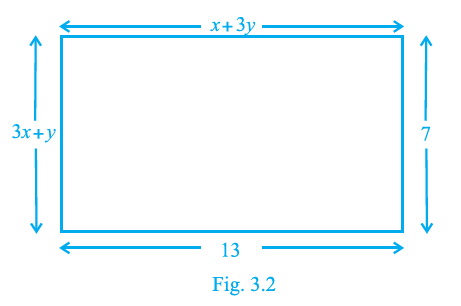Solution:

Using property of rectangle,

We know that,

Lengths are equal,

i.e., CD = AB

Hence, x + 3y = 13 …(i)

Hence, 3x + y = 7 …(ii)

On multiplying Eq. (ii) by 3 and then subtracting Eq. (i),

We get,

8x = 8

So, x = 1

On substituting x = 1 in Eq. (i),

We get,

y = 4

Therefore, the required values of x and y are 1 and 4, respectively.

## Exercise 3.4

1. Graphically, solve the following pair of equations:

2x + y = 6

2x y + 2 = 0

Find the ratio of the areas of the two triangles formed by the lines representing these equations with the x-axis and the lines with the y-axis.

Solution:

Given equations are 2x + y = 6 and 2x – y + 2 = 0

Table for equation 2x + y – 6 = 0, for x = 0, y = 6, for y = 0, x = 3.

 x 0 3 y 6 0

Table for equation 2x – y + 2 = 0, for x = 0, y = 2, for y = 0,x = – 1

 x 0 -1 y 2 0

Let A1 and A2 represent the areas of triangles ACE and BDE respectively.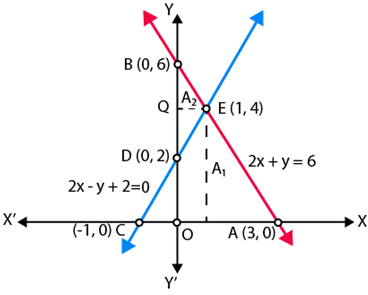Let, Area of triangle formed with x -axis = T1

T1 = Area of △ACE = ½ × AC × PE

T1 = ½ × 4 × 4 = 8

And Area of triangle formed with y – axis = T2

T1 = Area of △BDE = ½ × BD × QE

T1 = ½ × 4 × 1 = 2

T1:T2 = 8:2 = 4:1

Hence, the pair of equations intersect graphically at point E(1,4)

i.e., x = 1 and y = 4.

2. Determine, graphically, the vertices of the triangle formed by the lines

y = x, 3y = x, x + y = 8

Solution:

Given linear equations are

y = x …(i)

3y = x …(ii)

and x + y = 8 …(iii)

Table for line y = x,

 x 0 1 2 y 0 1 2

Table for line x = 3y,

 x 0 3 6 y 0 1 2

Table for line x + y = 8

 x 0 4 8 y 8 4 0

Plotting the points A (1, 1), B(2,2), C (3, 1), D (6, 2), we get the straight lines AB and CD.

Similarly, plotting the point P (0, 8), Q(4, 4) and R(8, 0), we get the straight line PQR.

AB and CD intersects the line PR on Q and D, respectively.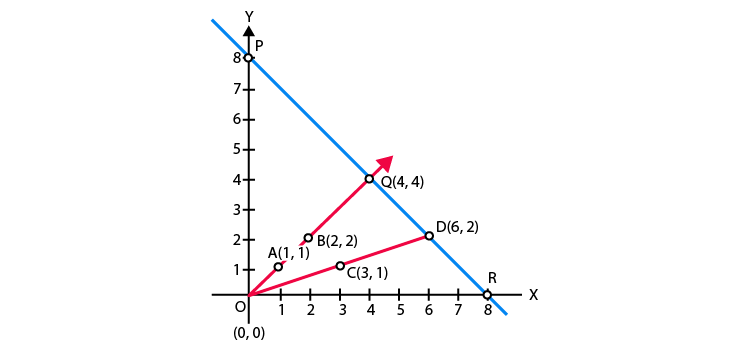So, △OQD is formed by these lines. Hence, the vertices of the △OQD formed by the given lines are O(0, 0), Q(4, 4) and D(6,2).

3. Draw the graphs of the equations x = 3, x = 5 and 2x y – 4 = 0. Also find the area of the quadrilateral formed by the lines and the x–axis.

Solution:

Given equation of lines x = 3, x = 5 and 2x-y-4 = 0.

Table for line 2x – y – 4 = 0

 x 0 2 y -4 0

Plotting the graph, we get,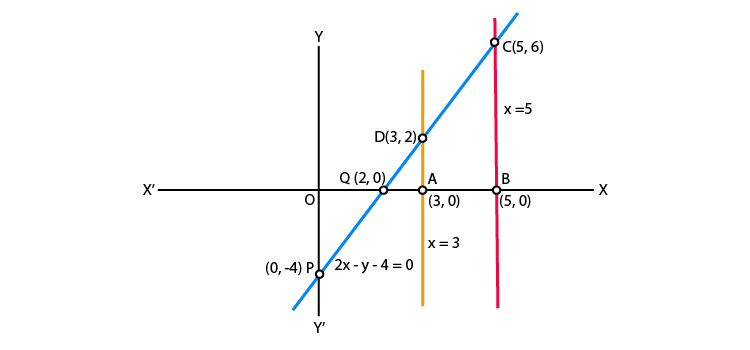From the graph, we get,

AB = OB-OA = 5-3 = 2

BC = 6

Thus, quadrilateral ABCD is a trapezium, then,

Area of Quadrileral ABCD = ½ × (distance between parallel lines) = ½ × (AB) × (AD + BC)

= 8 sq units

4. The cost of 4 pens and 4 pencil boxes is Rs 100. Three times the cost of a pen is Rs 15 more than the cost of a pencil box. Form the pair of linear equations for the above situation. Find the cost of a pen and a pencil box.

Solution:

Let the cost of a pen and a pencil box be Rs x and Rs y respectively.

According to the question,

4x + 4y = 100

Or x + y = 25 …(i)

3x = y + 15

Or 3x-y = 15 …(ii)

On adding Equation (i) and (ii), we get,

4x = 40

So, x = 10

Substituting x = 10, in Eq. (i) we get

y = 25-10 = 15

Hence, the cost of a pen = Rs. 10

The cost of a pencil box = Rs. 15

5. Determine, algebraically, the vertices of the triangle formed by the lines

3x y = 3

2x – 3y = 2

x + 2y = 8

Solution:

3x – y = 2 …(i)

2x -3y = 2 …(ii)

x + 2y = 8 …(iii)

Let the equations of the line (i), (ii) and (iii) represent the side of a ∆ABC.

On solving (i) and (ii),

We get,

[First, multiply Eq. (i) by 3 in Eq. (i) and then subtract]

(9x-3y)-(2x-3y) = 9-2

7x = 7

x = 1

Substituting x=1 in Eq. (i), we get

3×1-y = 3

y = 0

So, the coordinate of point B is (1, 0)

On solving lines (ii) and (iii),

We get,

[First, multiply Eq. (iii) by 2 and then subtract]

(2x + 4y)-(2x-3y) = 16-2

7y = 14

y = 2

Substituting y=2 in Eq. (iii), we get

x + 2 × 2 = 8

x + 4 = 8

x = 4

Hence, the coordinate of point C is (4, 2).

On solving lines (iii) and (i),

We get,

[First, multiply in Eq. (i) by 2 and then add]

(6x-2y) + (x + 2y) = 6 + 8

7x = 14

x = 2

Substituting x=2 in Eq. (i), we get

3 × 2 – y = 3

y = 3

So, the coordinate of point A is (2, 3).

Hence, the vertices of the ∆ABC formed by the given lines are as follows,

A (2, 3), B (1, 0) and C (4, 2).

6. Ankita travels 14 km to her home partly by rickshaw and partly by bus. She takes half an hour if she travels 2 km by rickshaw, and the remaining distance by bus. On the other hand, if she travels 4 km by rickshaw and the remaining distance by bus, she takes 9 minutes longer. Find the speed of the rickshaw and of the bus.

Solution:

Let the speed of the rickshaw and the bus are x and y km/h, respectively.

Now, she has taken time to travel 2 km by rickshaw, t1 = (2/x) hr

Speed = distance/ time

she has taken time to travel remaining distance i.e., (14 – 2) = 12km

By bus t2 = (12/y) hr

By first condition,

t1 + t2 = ½ = (2/x) + (12/y) … (i)

Now, she has taken time to travel 4 km by rickshaw, t3 = (4/x) hr

and she has taken time to travel remaining distance i.e., (14 – 4) = 10km, by bus = t4 = (10/y) hr

By second condition,

t3 + t4 = ½ + 9/60 = ½ + 3/20

(4/x) + (10/y) = (13/20) …(ii)

Let (1/x) = u and (1/y) = v

Then Equations. (i) and (ii) becomes

2u + 12v = ½ …(iii)

4u + 10v = 13/20…(iv)

[First, multiply Eq. (iii) by 2 and then subtract]

(4u + 24v) – (4u + 10v) = 1–13/20

14v = 7/20

v = 1/40

Substituting the value of v in Eq. (iii),

2u + 12(1/40) = ½

2u = 2/10

u = 1/10

x = 1/u = 10km/hr

y = 1/v = 40km/hr

Hence, the speed of rickshaw = 10 km/h

And the speed of bus = 40 km/h.

Also, BYJU’S is providing Class 10th Maths NCERT Solutions, notes, exemplar books, question papers and some preparation tips and tricks, so that students can score good marks in Maths subject. They are also suggested to solve sample papers and previous year question papers to get through the idea of question pattern and marking scheme for the chapter, Pair of Linear Equations in Two Variables.

The exemplar comes with detailed explanations to help students grasp the core concepts and get the right insight into how the problems should be handled. With our Exemplar, students will be able to study smartly, develop better math skills and ultimately be prepared to solve any type of question that may be asked in the exams. Get updated materials to learn from us and download BYJU’S-The Learning App to have an experience of a new method of learning with the help of educational videos clearing the concepts of Maths topics in a visual way.

## Frequently Asked Questions on NCERT Exemplar Solutions for Class 10 Maths Chapter 3

### What are the main topics discussed in NCERT Exemplar Solutions for Class 10 Maths Chapter 3?

The topics covered in Chapter 3 for Exemplar problems and solutions are:
1. Algebraic and geometrical representation of Pair of Linear Equations in Two Variables
2. Graphical methods of solving a pair of Linear equations
3. Algebraic methods of solving a pair of Linear equations such as substitution method, elimination method, cross-multiplication method
4. Reducing the pair of equations into a linear form
These topics are explained in a simple language to help students score well in the board exams irrespective of their intelligence quotient.

### What is the substitution method according to NCERT Exemplar Solutions for Class 10 Maths Chapter 3?

The substitution method is the algebraic method to solve simultaneous linear equations. As the word says, in this method, the value of one variable from one equation is substituted in the other equation. In this way, a pair of the linear equation gets transformed into one linear equation with only one variable, which can then easily be solved.

### Does NCERT Exemplar Solutions for Class 10 Maths Chapter 3 help you to score well in the board exams?

Students should first solve the easier problems and then move on to the problems of higher level difficulty. After completing each exercise, students will be able to analyse the areas in which they are lagging behind. By practising the weaker concepts numerous times, students will be able to perform well in the board exams. Short cut tips are also highlighted to help students understand the easier way of solving complex problems effortlessly./div>